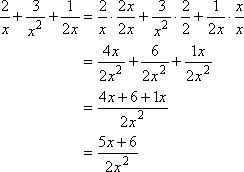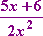Introduction
(page 1 of 3)Addition and subtraction are the hardest things you'll be doing with rational expressions because, just like with regular fractions, you'll have to convert to common denominators. Everything you hated about adding fractions, you're going to hate worse with rational expressions. But stick with me; you can get through this!

Let's refresh by looking at an example with regular fractions:

• Simplify the following:
•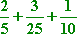To find the common denominator, I first need to find the least common multiple (LCM) of the three denominators. (For old folks like me, whenever you see "LCM", think "LCD", or "lowest common denominator". In this context, they're pretty much the same thing.) There are at least a couple ways of doing this. You could use the "listing" method, where you list the multiples of the three denominators, until you find a number that is in all three lists, like this:

5:  5, 10, 15, 20, 25, 30, 35, 40, 45, 50, 55,...

25:  25, 50, 75, 100, 125, 150, 175, 200,...

10:  10, 20, 30, 40, 50, 60, 70, 80, 90, 100,...

The first multiple to occur in all three lists is 50, so this will be the common denominator.

Another method you could use for finding the common denominator is the factor method. It works by finding the prime factorization of each denominator, and then using a chart to find the factors needed for the common denominator. It looks like this: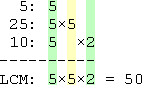In either case, the common denominator will be 50. To convert each fraction to the common denominator, you multiply each denominator by what it needs in order to turn it into "50". For instance, in the 2/5, the denominator needs to be multiplied by 10, since 10×5 = 50. To keep things fair, you multiply the top by 10 as well. This is because 10/10 = 1, and multiplying things by 1 doesn't actually change them. So you get: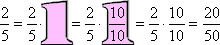Converting the other fractions, you get: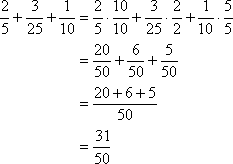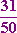The process works similarly for rationals.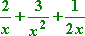To find the common denominator, I need to find the least common multiple of x, x2, and 2x. In the previous problem, I used the "listing" method to find the common denominator. But in this case, I've got numbers and variables, so just multiplying by numbers is not going to work. To find the common denominator above, all I had to do was multiply each denominator by 1, then 2, then 3, then 4,... and so on, until I found a match. But what should I multiply the variables by? So "listing" won't work for rationals. I'll have to use the factor method instead. Here's what I get: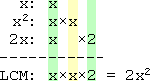My common denominator will be 2x2. To convert the "2/x" to the common denominator, I will need to multiply by 2x/2x, since the denominator already has one copy of x but needs a 2 and another x: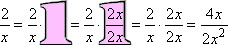Similarly, for the 3/x2, I will multiply by 2/2; and for the 1/2x, I will multiply by x/x. This gives me: